# I3ck/cppOpt

Fetching contributors…Cannot retrieve contributors at this time
232 lines (179 sloc) 6.32 KB

# cppOpt

A header-only C++ library which allows the numerical optimisation of any given problem, function, program or you-name-it
without knowing the "function" of the problem simply by smartly testing certain values

## version 0.3.0

Bascially the entire structure of the library has been changed. The performance has been optimised as well, so a switch to the new version might be worth it.
Otherwise simply stick to earlier versions of `cppOpt`.

## optimise anything!

optimise any given problem by providing an `std::function`

```auto toOptimize = [](OptCalculation<double>& optCalculation) {
optCalculation.result = YOUR CODE COMES HERE
};```

for optimising x^2 you could write:

```auto toOptimize = [](OptCalculation<double>& optCalculation) {
optCalculation.result = pow(optCalculation.get_parameter("X"), 2);
};```

you can even run other programs:

```auto toOptimize = [](OptCalculation<double>& optCalculation) {
std::string parameter1 = optCalculation.get_parameter("X").to_string();
std::string parameter2 = optCalculation.get_parameter("Y").to_string();

system(( "someExecuteable -p1 " + parameter1 + " -p2 " + parameter2).c_str() );
optCalculation.result = parse_generated_output_file();
}```

## define your parameters and boundaries

```OptBoundaries<T> optBoundaries;

## minimize, maximize, approach or diverge

```enum class OptTarget {
MINIMIZE,
MAXIMIZE,
APPROACH,
DIVERGE
};```

## templated

use any of these:

```<float>
<double>
<long double>```

## create your coordinator

```// the bool flag decides whether multithreading is active
OptCoordinator<double, false> coordinator(
maxCalculations,
toOptimize,
optTarget,
targetValue);```

```coordinator.add_child(make_unique<OptSimulatedAnnealing<double>>(...))

you can add any number of child algorithms and run these in parallel. Just make sure your calculation callback is thread-safe.

## go!

```coordinator.run_optimisation(); // if single threaded

## aborting early

you can also define when to abort the optimisation, in case the value is good enough

`coordinator.enable_early_abort(13.37);`

## logging / outputting results

add logging by via your calculate function

```auto toOptimize = [](OptCalculation<double>& optCalculation) {
optCalculation.result = YOUR CODE COMES HERE

//also log the result
cout << optCalculation.to_string_values() << " =>\t" << optCalculation.result << endl;
};```
``````2.162039 4.674414 =>	4.67441
-0.185813 0.034526 =>	0.0345263
-1.505135 2.265430 =>	2.26543
-4.193620 17.586445 =>	17.5864
-2.887129 8.335513 =>	8.33551
-0.469162 0.220113 =>	0.220113
-3.301420 10.899376 =>	10.8994
-2.083137 4.339458 =>	4.33946
2.035803 4.144495 =>	4.1445
2.998251 8.989506 =>	8.98951
0.830960 0.690495 =>	0.690495
``````

you can retrieve the best value once the optimisation is done

```OptCalculation<double> best = coordinator.get_best_calculation();
cout << best.to_string_header() << endl;
cout << best.to_string_values() << endl;```

## examples

Please refer to the `examples/` folder for well documented and currently compiling usage examples.

### optimising x*x [-5.0:+5.0] with simulated annealing

note that the optimiser doesn't "know" that the function actually is x*x

#### minimizing: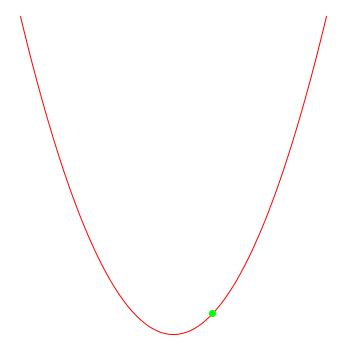#### maximizing: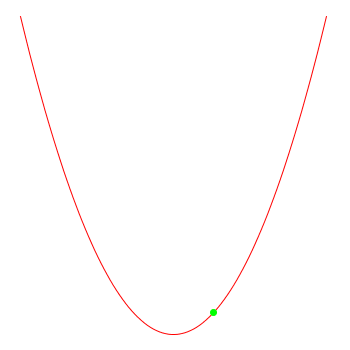#### approaching 3.0: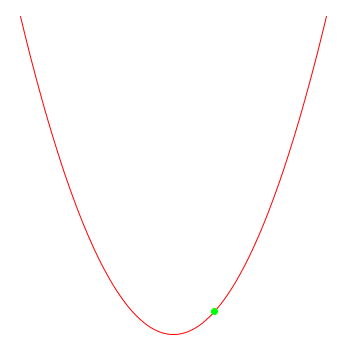### optimising the rastrigrin function with simulated annealing

http://en.wikipedia.org/wiki/Rastrigin_function

#### one-dimensional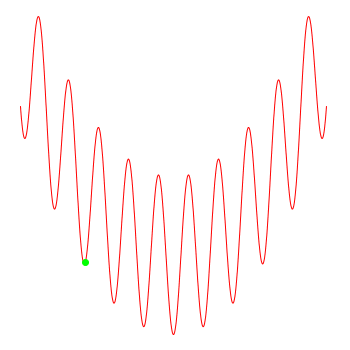#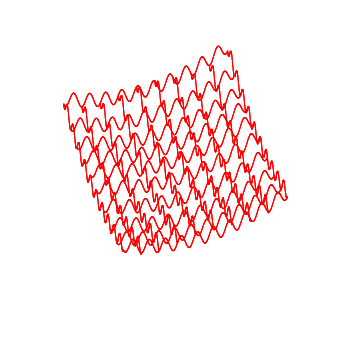usage

## configuration

open up `inc/config.h` to enable/disable DEBUG output

`//#define DEBUG`

## building the examples / tests

`cmake .`
`make`

## including

directly include `cppOpt.h` which includes all required headers or choose the header files you want to use

## testing

after using `cmake` and `make` check `bin/` for the `test_xyz` executeables
since the tests check whether the algorithms reach the wanted values, it's possible that the tests might fail in some cases (due to the random nature of the algorithms). Just restart the test in such a case.
Only if the test fails often there's an actual bug

# algorithms explained / referenced

## simulated annealing

http://en.wikipedia.org/wiki/Simulated_annealing

## threshold accepting

http://comisef.wikidot.com/concept:thresholdaccepting

## great deluge

http://en.wikipedia.org/wiki/Great_Deluge_algorithm

## evolutionary

https://en.wikipedia.org/wiki/Evolutionary_algorithm

# contribute

feel free to open issues if you'd like to have new algorithms added, would like to have a new feature or found a bug

You can’t perform that action at this time.